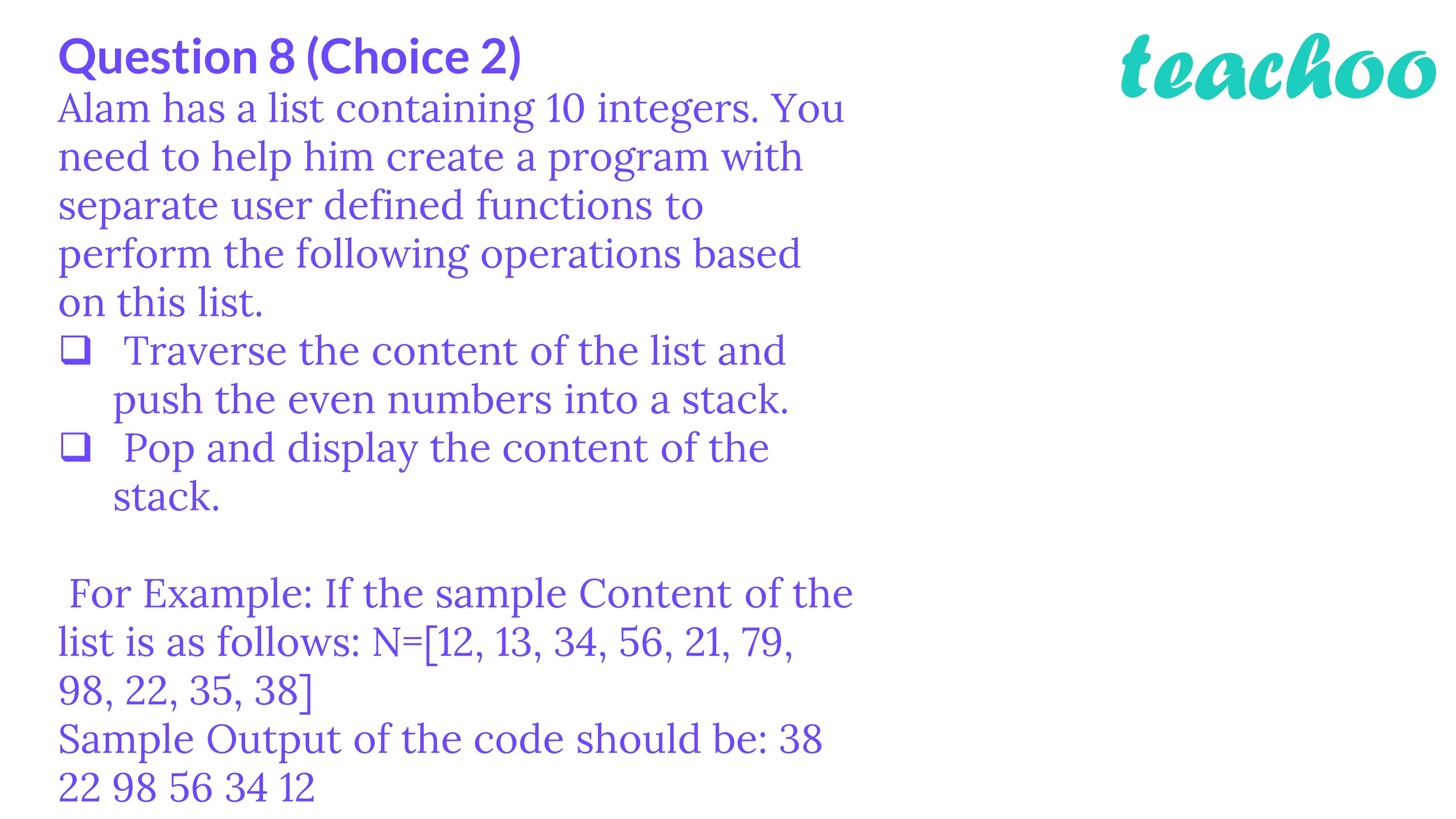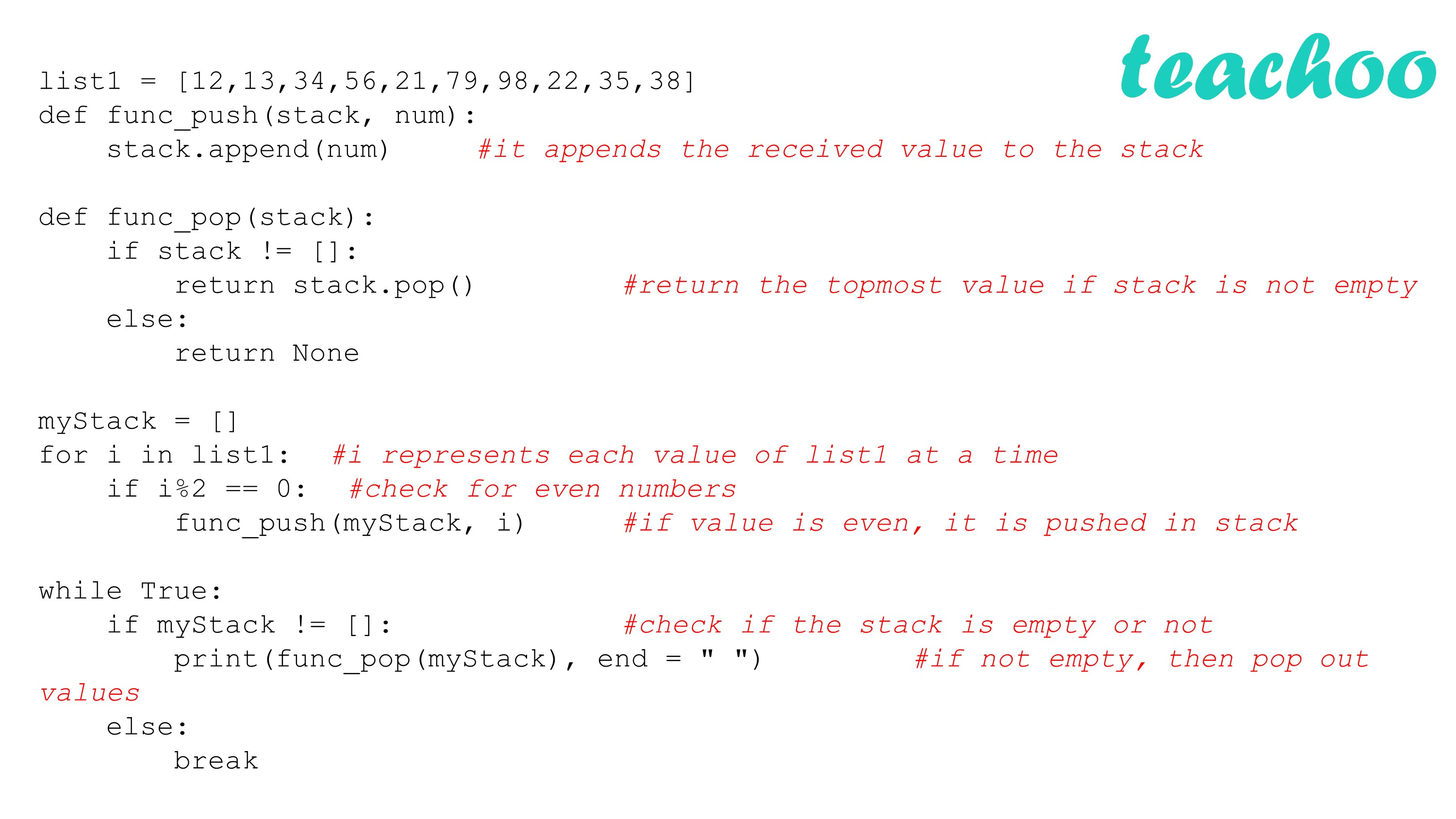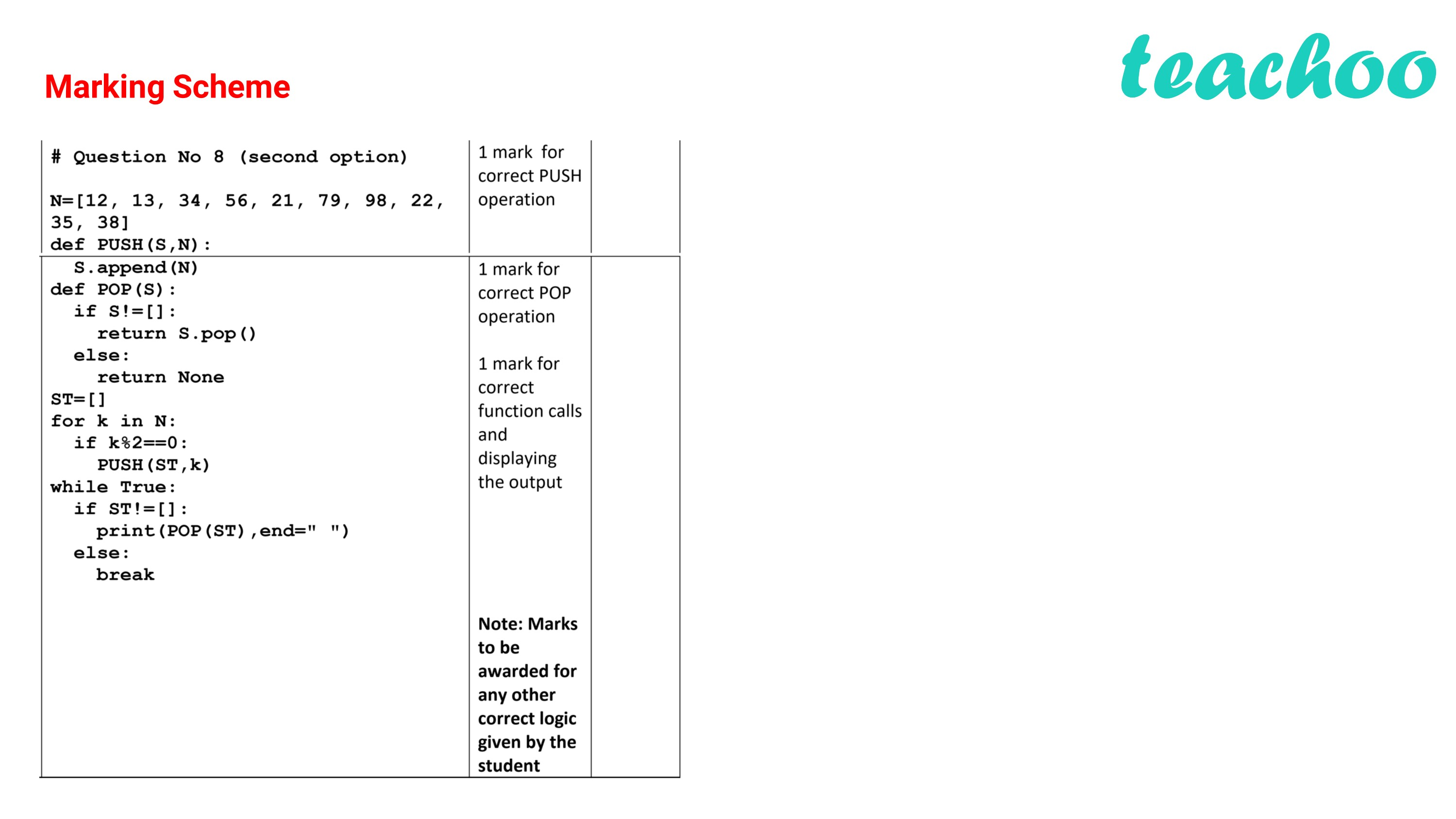CBSE Class 12 Sample Paper for 2022 Boards [Term 2] - Computer Science

Computer Science - Class 12
Solutions to CBSE Sample Paper - Computer Science Class 12

Alam has a list containing 10 integers. You need to help him create a program with separate user defined functions to perform the following operations based on this list.

• Traverse the content of the list and push the even numbers into a stack.
• Pop and display the content of the stack.

For Example: If the sample Content of the list is as follows: N=[12, 13, 34, 56, 21, 79, 98, 22, 35, 38]

Sample Output of the code should be: 38 22 98 56 34 12

Program:

``` list1 = [ 12 , 13 , 34 , 56 , 21 , 79 , 98 , 22 , 35 , 38 ] ```

``` def func_push (stack, num):  ```

```     stack.append(num)       #it appends the received value to the stack ```

``` def func_pop (stack): ```

```     if stack != []: ```

```         return stack.pop()      #return the topmost value if stack is not empty ```

```     else: ```

```         return None ```

```       ```

``` myStack = [] ```

``` for i in list1:     #i represents each value of list1 at a time ```

```     if i% 2 == 0 :        #check for even numbers ```

```         func_push(myStack, i)       #if value is even, it is pushed in stack ```

```   ```

``` while True: ```

```     if myStack != []:       #check if the stack is empty or not ```

```         print(func_pop(myStack), end = " " )     #if not empty, then pop out values ```

```     else: ```

```         break ```

Output:

``` 38 22 98 56 34 12 `````` ```

Get live Maths 1-on-1 Classs - Class 6 to 12